# How to set the Location of the TextBox in C#?

In Windows forms, TextBox plays an important role. With the help of TextBox, the user can enter data in the application, it can be of a single line or of multiple lines. In TextBox, you are allowed to set the coordinates of the upper-left corner of the TextBox control relative to the upper-left corner of its container with the help of Location property. In Windows form, you can set this property in two different ways:

1. Design-Time: It is the simplest way to set the Location property of the TextBox as shown in the following steps:

• Step 1: Create a windows form as shown in the below image:
Visual Studio -> File -> New -> Project -> WindowsFormApp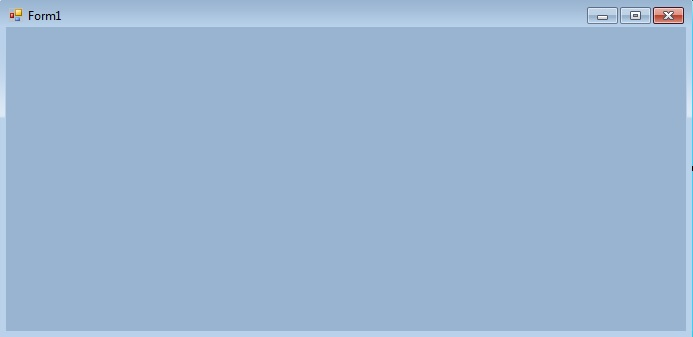• Step 2: Drag the TextBox control from the ToolBox and Drop it on the windows form. You can place TextBox anywhere on the windows form according to your need. As shown in the below image: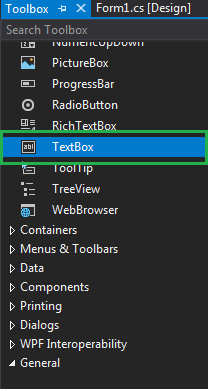• Step 3: After drag and drop you will go to the properties of the TextBox control to set the Location property of the TextBox. As shown in the below image: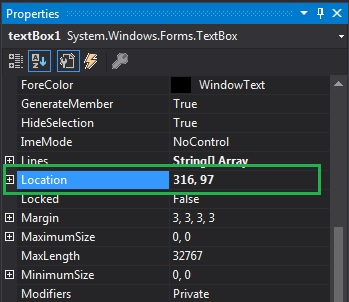Output: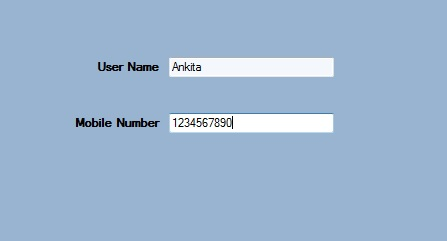2. Run-Time: It is a little bit trickier than the above method. In this method, you can set the Location property of the TextBox programmatically with the help of given syntax:

`public System.Drawing.Point Location { get; set; }`

Here, Point is used to represent the upper-left corner of the control relative to the upper-left corner of its container. Following steps are used to set the Location property of the TextBox:

• Step 1 : Create a textbox using the TextBox() constructor provided by the TextBox class.
```// Creating textbox
TextBox Mytextbox = new TextBox();
```
• Step 2 : After creating TextBox, set the Location property of the TextBox provided by the TextBox class.
```// Set Location property
Mytextbox1.Location = new Point(187, 51);
```
• Step 3 : And last add this textbox control to from using Add() method.
```// Add this textbox to form
```

Example:

 `using` `System; ` `using` `System.Collections.Generic; ` `using` `System.ComponentModel; ` `using` `System.Data; ` `using` `System.Drawing; ` `using` `System.Linq; ` `using` `System.Text; ` `using` `System.Threading.Tasks; ` `using` `System.Windows.Forms; ` ` `  `namespace` `my { ` ` `  `public` `partial` `class` `Form1 : Form { ` ` `  `    ``public` `Form1() ` `    ``{ ` `        ``InitializeComponent(); ` `    ``} ` ` `  `    ``private` `void` `Form1_Load(``object` `sender, EventArgs e) ` `    ``{ ` `        ``// Creating and setting the properties of Lable1 ` `        ``Label Mylablel1 = ``new` `Label(); ` `        ``Mylablel1.Location = ``new` `Point(96, 54); ` `        ``Mylablel1.Text = ``"Enter Name"``; ` `        ``Mylablel1.AutoSize = ``true``; ` `        ``Mylablel1.BackColor = Color.LightGray; ` ` `  `        ``// Add this label to form ` `        ``this``.Controls.Add(Mylablel1); ` ` `  `        ``// Creating and setting the properties of TextBox1 ` `        ``TextBox Mytextbox1 = ``new` `TextBox(); ` `        ``Mytextbox1.Location = ``new` `Point(187, 51); ` `        ``Mytextbox1.BackColor = Color.LightGray; ` `        ``Mytextbox1.AutoSize = ``true``; ` `        ``Mytextbox1.Name = ``"text_box1"``; ` `        ``Mytextbox1.Margin = ``new` `Padding(5, 5, 5, 5); ` ` `  `        ``// Add this textbox to form ` `        ``this``.Controls.Add(Mytextbox1); ` ` `  `        ``// Creating and setting the properties of Lable1 ` `        ``Label Mylablel2 = ``new` `Label(); ` `        ``Mylablel2.Location = ``new` `Point(96, 102); ` `        ``Mylablel2.Text = ``"Enter Area"``; ` `        ``Mylablel2.AutoSize = ``true``; ` `        ``Mylablel2.BackColor = Color.LightGray; ` ` `  `        ``// Add this label to form ` `        ``this``.Controls.Add(Mylablel2); ` ` `  `        ``// Creating and setting the properties of TextBox2 ` `        ``TextBox Mytextbox2 = ``new` `TextBox(); ` `        ``Mytextbox2.Location = ``new` `Point(187, 99); ` `        ``Mytextbox2.BackColor = Color.LightGray; ` `        ``Mytextbox2.AutoSize = ``true``; ` `        ``Mytextbox2.Name = ``"text_box2"``; ` ` `  `        ``// Add this textbox to form ` `        ``this``.Controls.Add(Mytextbox2); ` `    ``} ` `} ` `} `

Output: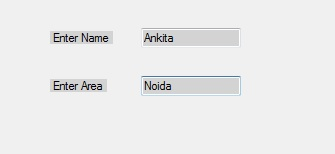My Personal Notes arrow_drop_upCheck out this Author's contributed articles.

If you like GeeksforGeeks and would like to contribute, you can also write an article using contribute.geeksforgeeks.org or mail your article to contribute@geeksforgeeks.org. See your article appearing on the GeeksforGeeks main page and help other Geeks.

Please Improve this article if you find anything incorrect by clicking on the "Improve Article" button below.

Article Tags :

1

Please write to us at contribute@geeksforgeeks.org to report any issue with the above content.